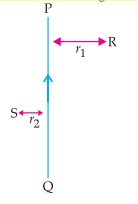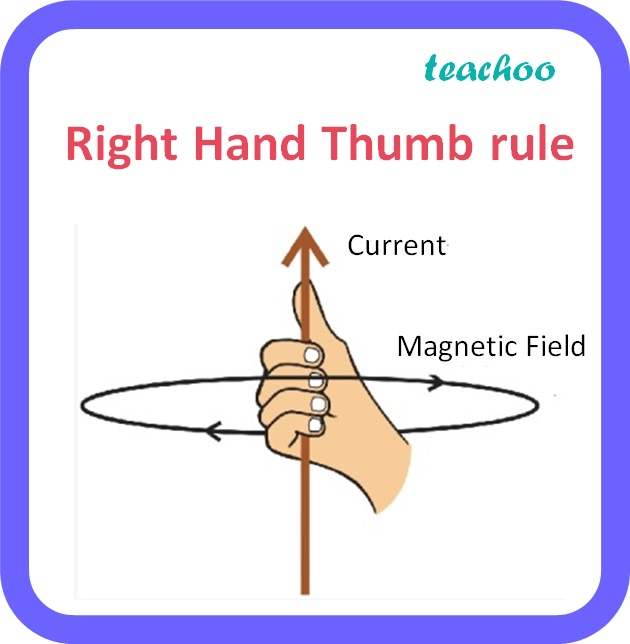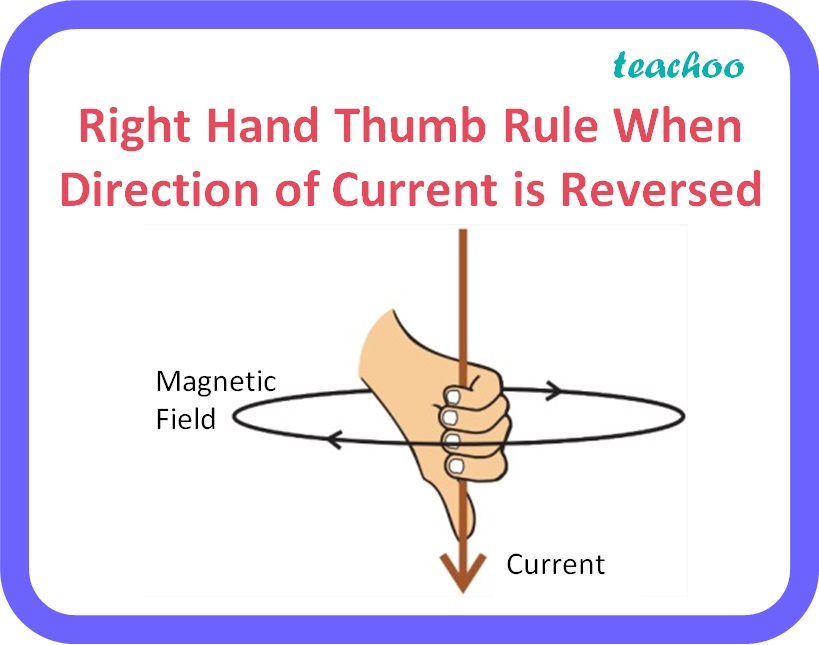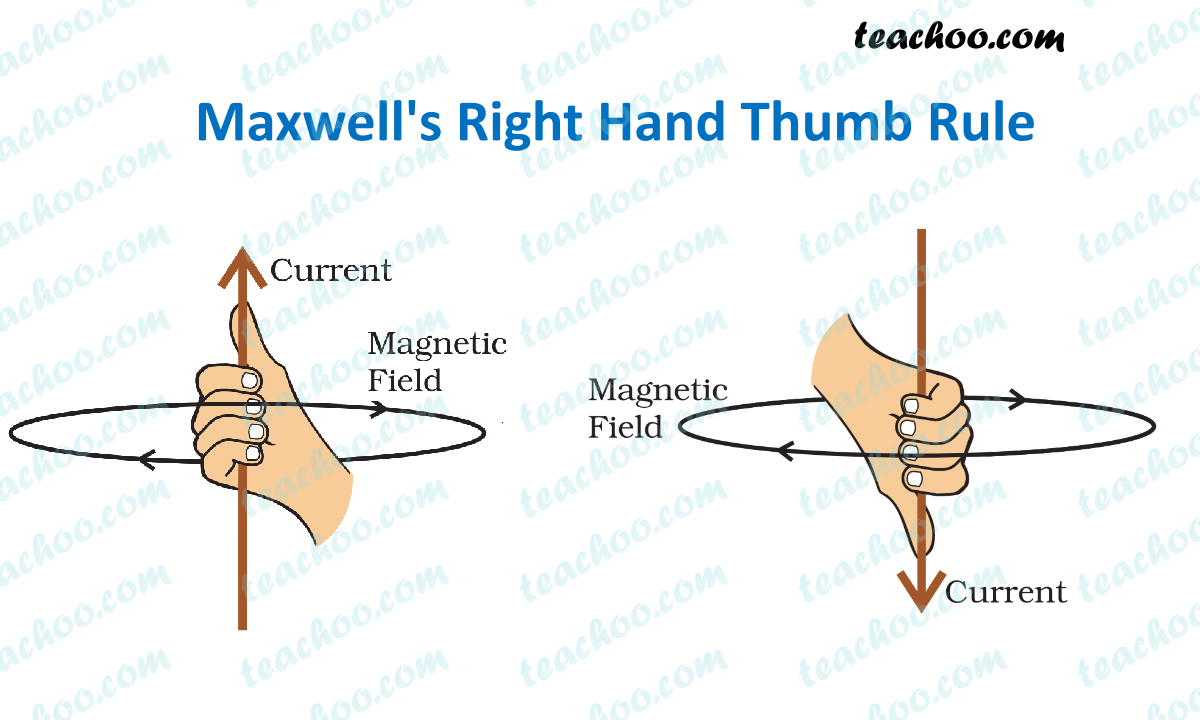Past Year - 5 Mark Questions

Class 10
Chapter 13 Class 10 - Magnetic Effects of Electric Current

## PQ is a current carrying conductor in the plane of the paper as shown in the figure below.## (iv) Explain the rule that is used to find the direction of the magnetic field for a straight current carrying conductor.

(i)According to right hand thumb rule, the magnetic field lines produced is:

• Into the plane of the paper in at R
• Out of it at S

(ii)

Magnetic field strength for a straight current carrying conductor is inversely proportional to the distance from the wire.

So, as distance from the conductor decreases, magnetic field strength increases.

Since r 2 < r 1

• Magnetic field strength at S will be greater than magnetic field strength at R.

(iii)If the polarity of the battery connected to the wire is reversed, then the direction of current through the conductor is also reversed ie., current will now flow from top to bottom and the direction of magnetic field lines will also be reversed.

Now, the magnetic field lines will be:

• Out of the plane of the paper at R
• Into the plane of the paper in at S

(iv)

Right Hand Thumb rule is used to find the direction of the magnetic field for a straight current carrying conductor.It states that, if we point the thumb of our right hand in the direction of the current, then the direction in which our fingers curl gives the direction of the magnetic field.

Learn in your speed, with individual attention - Teachoo Maths 1-on-1 Class